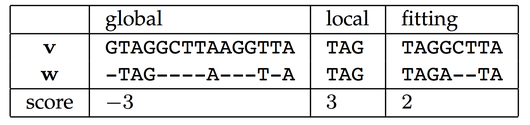# Glossary

## Fitting alignment

A fitting alignment of a string $s$ against another string $t$ is defined as an alignment of $s$ with a substring $t'$ of $t$. As usual in alignment problems, we aim to find a minimum-score fitting alignment across all possible such substrings $t'$ of $t$, where the particular alignment score used may vary.

Note that we are allowed to use a substring only of $t$ in the alignment, not $s$. This makes the problem of finding a fitting alignment a hybrid of global and local alignment. See the figure below for a comparison of global, local, and fitting alignments of the strings $v = \textrm{GTAGGCTTAAGGTTA}$ and $w = \textrm{TAGATA}$, where $w$ is aligned against a substring of $v$ in the fitting alignment.The most common biological application of fitting alignments arises when $s$ represents a known motif that we are hoping to match against a larger genetic string $t$ (with some errors due to small-scale mutations). For example, $s$ may represent a known gene that we wish to locate with some changes within a genome $t$; alternatively, $s$ could encode a known domain that we are comparing against a newly discovered protein.